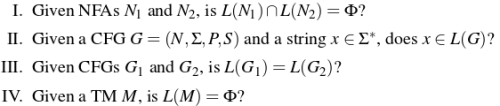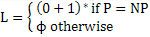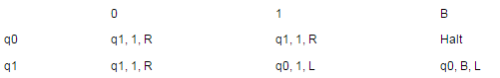Courses

# Turing Machines & Undecidability - 2

## 20 Questions MCQ Test GATE Computer Science Engineering(CSE) 2022 Mock Test Series | Turing Machines & Undecidability - 2

Description
This mock test of Turing Machines & Undecidability - 2 for Computer Science Engineering (CSE) helps you for every Computer Science Engineering (CSE) entrance exam. This contains 20 Multiple Choice Questions for Computer Science Engineering (CSE) Turing Machines & Undecidability - 2 (mcq) to study with solutions a complete question bank. The solved questions answers in this Turing Machines & Undecidability - 2 quiz give you a good mix of easy questions and tough questions. Computer Science Engineering (CSE) students definitely take this Turing Machines & Undecidability - 2 exercise for a better result in the exam. You can find other Turing Machines & Undecidability - 2 extra questions, long questions & short questions for Computer Science Engineering (CSE) on EduRev as well by searching above.
QUESTION: 1

### Let < M > be the encoding of a Turing machine as a string over {0, 1}. Let L = { < M > |M is a Turing machine that accepts a string of length 2014 }. Then, L is

Solution:

There are finite number of strings of length ‘2014’. So, a turing machine will take the input string of length ‘2014’ and test it.
If, input string is present in the language then turing machine will halt in final state . But, if turing machine is unable to accept the input string then it will halt in non-final state or go in an infinite loop and never halt.

Thus, ‘L’ is undecidable and recursively enumerable .

Please comment below if you find anything wrong in the above post.

QUESTION: 2

### Which of the following problems is undecidable?

Solution:

Context free grammar is not closed under ambiguity.A set is closed under an operation means when we operate an element of that set with that operator we get an element from that set. Here, context free grammar generates a context free language and set of all context free languages is also a set. But, ambiguity is not an operation and hence we can never say that CFG is closed under ambiguity. Thus, problem mentioned in option (A) is undecidable. Please comment below if you find anything wrong in the above post.

QUESTION: 3

### Consider three decision problems P1, P2 and P3. It is known that P1 is decidable and P2 is undecidable. Which one of the following is TRUE?

Solution:

Background: In computational complexity theory, a decision problem has only two possible outputs yes or no. A decision problem is said to be decidable if there exists an effective method or algorithm that returns a correct yes/no answer to that problem. A decision problem is said to be undecidable if there does not exist a single algorithm that always lead to a correct yes/no solution. In terms of reducibility: A ≤p B denotes A is a decision problem that is reducible to B in polynomial time p. This simply means that A’s instance can be transformed into B’s instance and following the solution of B we can get a solution for the problem A. So here we can draw some conclusions:

1. If A ≤p B and B is decidable then A is also decidable. This is because if there exists a specific algorithm for solving B and we can also reduce A to B then we can have a solution of A as well. Hence A is decidable.
However the reverse is not true i.e. if A ≤p B and A is decidable then B is also decidable because A can have an algorithm existing for its correct solution but might be the case that B does not.
2. If A ≤p B and A is undecidable then B is also undecidable. This is because if A is undecidable even when it can be reduced to B that simply reflects even B cannot provide an algorithm by which we can solve B and hence A. So decision problem B is also undecidable.

However the reverse is not true here as well i.e. if A ≤p B and B is undecidable then A is also undecidable because there might exist an algorithm for A that can provide a solution to A. Using the above stated conclusions we can say that option 1, 2 and 4 are false and option 3 is true.

Option 1: P1 ≤p P3 and given P1 is decidable gives no conclusion for P3.
Option 2: P3 ≤p P2 and given P2 is undecidable gives no conclusion for P3.
Option 3: P2 ≤p P3 and given P2 is undecidable gives conclusion for P3 to be undecidable.
Option 4: P3 ≤p P2’s complement and given P2 is undecidable therefore P2’s complement is also undecidable gives no conclusion for P3.

QUESTION: 4

Consider two languages L1 and L2 each on the alphabet ∑. Let f : ∑ → ∑ be a polynomial time computable bijection such that (∀ x) [x ∈ L1 iff f(x) ∈ L2]. Further, let f-1 be also polynomial time computable. Which of the following CANNOT be true?

Solution:

We have one to one mapping for all instances of L1 to L2. L1 is given to be undecidable. Further L1 is polynomial time reducible to L2. (By given mapping). Now if L2 is decidable then there is algorithm to solve L2 in polytime. But then we can solve every instance of L1 in polytime, making L1 also decidable. Contradiction

QUESTION: 5

Consider the following problem X.

Given a Turing machine M over the input alphabet Σ, any state q of M And a word w∈Σ*, does the computation of M on w visit the state q?

Q. Which of the following statements about X is correct?

Solution:

This problem is a State Entry Problem. State entry problem can be reduced to halting problem.

We construct a turing machine M with final state ‘q’. We run a turing machine R (for state entry problem) with inputs : M, q, w .
We give ‘w’ as input to M.
If M halts in the final state ‘q’ then R accepts the input. So, the given problem is partially decidable. If M goes in an infinite loop then M can not output anything. So, R rejects the input. So, the given problem becomes undecidable.

Thus, option (B) is the answer.

Please comment below if you find anything wrong in the above post.

QUESTION: 6

Consider the following decision problems:

(P1) Does a given finite state machine accept a given string
(P2) Does a given context free grammar generate an infinite number of stings

Q. Which of the following statements is true?

Solution:
• A finite state machine always halts in final or non-final state.Therefore, problem P1 is decidable.
• We check if the context free language generates any string of length between n and (2n – 1). If so, context free language is infinite else it is finite.Therefore, problem P2 is decidable.  Thus, option (A) is correct. Please comment below if you find anything wrong in the above post.
QUESTION: 7

Consider the following statements:

1. The complement of every Turning decidable language is Turning decidable
2. There exists some language which is in NP but is not Turing decidable
3. If L is a language in NP, L is Turing decidable

Q. Which of the above statements is/are True?

Solution:

1 is true: Complement of Turing decidable is Turing Decidable.
3 is true. All NP problems are Turing decidable
2 is false: The definition of NP itself says solvable in polynomial time using non-deterministic Turing machine.

QUESTION: 8

Which of the following decision problems are undecidableSolution:

A problem is undecidable if there is no algorithm to find the solution for it. Problem give in III and IV have no solutions, so these are undecidable.

QUESTION: 9

Let L be a language and L' be its complement. Which one of the following is NOT a viable possibility?

Solution:

A) It is possible if L itself is NOT RE. Then L' will also not be RE.
B) Suppose there is a language such that turing machine halts on the input. The given language is RE but not recursive and its complement is NOT RE.
C) This is not possible because if we can write enumeration procedure for both languages and it's complement, then the language becomes recursive.
D) It is possible because L is closed under complement if it is recursive.   Thus, C is the correct choice.

QUESTION: 10

Let A ≤m B denotes that language A is mapping reducible (also known as many-to-one reducible) to language B. Which one of the following is FALSE?

Solution:
• A ≤m B means language A is mapping reducible to language B.Thus, A cannot be harder than B. Since, A can be reduced to B, instead of deciding A we can now decide B. So, the first three options are correct.
• As B is not recursively enumerable, it doesn't guarantee A is not recursively enumerable.Thus, if A ≤m B and B is not recursively enumerable then A is not recursively enumerable. Therefore, answer is D is correct

Please comment below if you find anything wrong in the above post.

QUESTION: 11

For S ∈ (0 + 1) * let d(s) denote the decimal value of s (e.g. d(101) = 5). Let L = {s ∈ (0 + 1)* d(s)mod5 = 2 and d(s)mod7 != 4}. Which one of the following statements is true?

Solution:

It is regular L1=d(s) mod 5 =2 is regular with 5 states L2=d(s) mod 7 =4 is regular with 7 states therefore L1 ^ L2' should be regular because regular grammar are closed under intersection and compliment

QUESTION: 12

Let L1 be a recursive language, and let L2 be a recursively enumerable but not a recursive language. Which one of the following is TRUE?

L1' --> Complement of L1
L2' --> Complement of L2

Solution:

Recursively enumerable languages are known as type-0 languages in the Chomsky hierarchy of formal languages. All regular, context-free, context-sensitive and recursive languages are recursively enumerable.
Recursive Languages are closed under complementation, but recursively enumerable are not closed under complementation.  If a languages L is recursively enumerable, then the complement of it is recursively enumerable if and only if  L is also recursive.  Since L2 is recursively enumerable, but not recursive, L2' is not recursively enumerable.

QUESTION: 13

L1 is a recursively enumerable language over Σ. An algorithm A effectively enumerates its words as w1, w2, w3, ... Define another language L2 over Σ Union {#} as {wi # wj : wi, wj ∈ L1, i < j}. Here # is a new symbol. Consider the following assertions.

S1 : L1 is recursive implies L2 is recursive
S2 : L2 is recursive implies L1 is recursive

Q. Which of the following statements is true ?

Solution:
QUESTION: 14

Nobody knows yet if P = NP. Consider the language L defined as follows :Q. Which of the following statements is true ?

Solution:

Answer is A. in both case(P = NP or P != NP) L is regular, so L is recursive. Thanks to Vikrant for the above explanation.

QUESTION: 15

A single tape Turing Machine M has two states q0 and q1, of which q0 is the starting state. The tape alphabet of M is {0, 1, B} and its input alphabet is {0, 1}. The symbol B is the blank symbol used to indicate end of an input string. The transition function of M is described in the following table.Q. The table is interpreted as illustrated below. The entry (q1, 1, R) in row q0 and column 1 signifies that if M is in state q0 and reads 1 on the current tape square, then it writes 1 on the same tape square, moves its tape head one position to the right and transitions to state q1. Which of the following statements is true about M ?

Solution:

Whenever B is given as a input, turing machine halts. This implies epsilon is only accepted when B occurs as an input.

In positive closure, epsilon is not present. So, Turing machine never halts in case of (0+1)+.

Thus, option (A) is correct.

Please comment below if you find anything wrong in the above post.

QUESTION: 16

Define languages L0 and L1 as follows :

L0 = {< M, w, 0 > | M halts on w}
L1 = {< M, w, 1 > | M does not halts on w}

Here < M, w, i > is a triplet, whose first component. M is an encoding of a Turing Machine, second component, w, is a string, and third component, i, is a bit. Let L = L0 ∪ L1. Which of the following is true ?

Solution:
QUESTION: 17

Which of the following is true?

Solution:
QUESTION: 18

For any two languages L1 and L2 such that L1 is context free and L2 is recursively enumerable but not recursive, which of the following is/are necessarily true?

1. L1' (complement of L1) is recursive
2. L2' (complement of L2) is recursive
3. L1' is context-free
4. L1' ∪ L2 is recursively enumerable

Solution:

1. L1' (complement of L1) is recursive is true L1 is context free. Every context free language is also recursive and recursive languages are closed under complement. 4. L1' ∪ L2 is recursively enumerable is true Since L1' is recursive, it is also recursively enumerable and recursively enumerable languages are closed under union. Recursively enumerable languages are known as type-0 languages in the Chomsky hierarchy of formal languages. All regular, context-free, context-sensitive and recursive languages are recursively enumerable. (Source: Wiki) 3. L1' is context-free: Context-free languages are not closed under complement, intersection, or difference. 2. L2' (complement of L2) is recursive is false: Recursively enumerable languages are not closed under set difference or complementation

QUESTION: 19

Let X be a recursive language and Y be a recursively enumerable but not recursive language. Let W and Z be two languages such that Y' reduces to W, and Z reduces to X' (reduction means the standard many-one reduction). Which one of the following statements is TRUE

Solution:

Since X is recursive and recursive language is closed under complement. So X’ is also recursive. Since  Z ≤ X’ is recursive. (Rule : if Z is reducible to X’ , and X’ is recursive, then Z is recursive.) Option (B) and (D) is eliminated. And Y is recursive enumerable but not recursive, so Y’ cannot be recursively enumerable. Since Y’ reduces to W. And we know complement of recursive enumerable is not recursive enumerable and therefore, W is not recursively enumerable. So Correct option is (C). Here Y’ is complement of Y and X’ is complement of X.

QUESTION: 20

Consider the following types of languages:

L1 Regular,
L2: Context-free,
L3: Recursive,
L4: Recursively enumerable.

Q. Which of the following is/are TRUE?

I. L3' U L4 is recursively enumerable
II. L2 U L3 is recursive
III. L1* U L2 is context-free
IV. L1 U L2' is context-free

Solution:

St 1: As L3 is Recursive and recursive languages are closed under complementation, L3’ will also be recursive. L3’ U L4 is also recursive as recursive languages are closed under union.
St 2: As L2 is Context- Free, it will be recursive as well. L2 U L3 is recursive because as recursive languages are closed under union.
St 3: L1* is regular because regular languages are closed under kleene –closure. L1* U L2 is context free as union of regular and context free is context free.
St 4: L2’ may or may not be context free because CFL are not closed under complementation. So it is not true. So I, II and III are correct.